Verify Rolles theorem

Chapter 5 Class 12 Continuity and Differentiability
Concept wise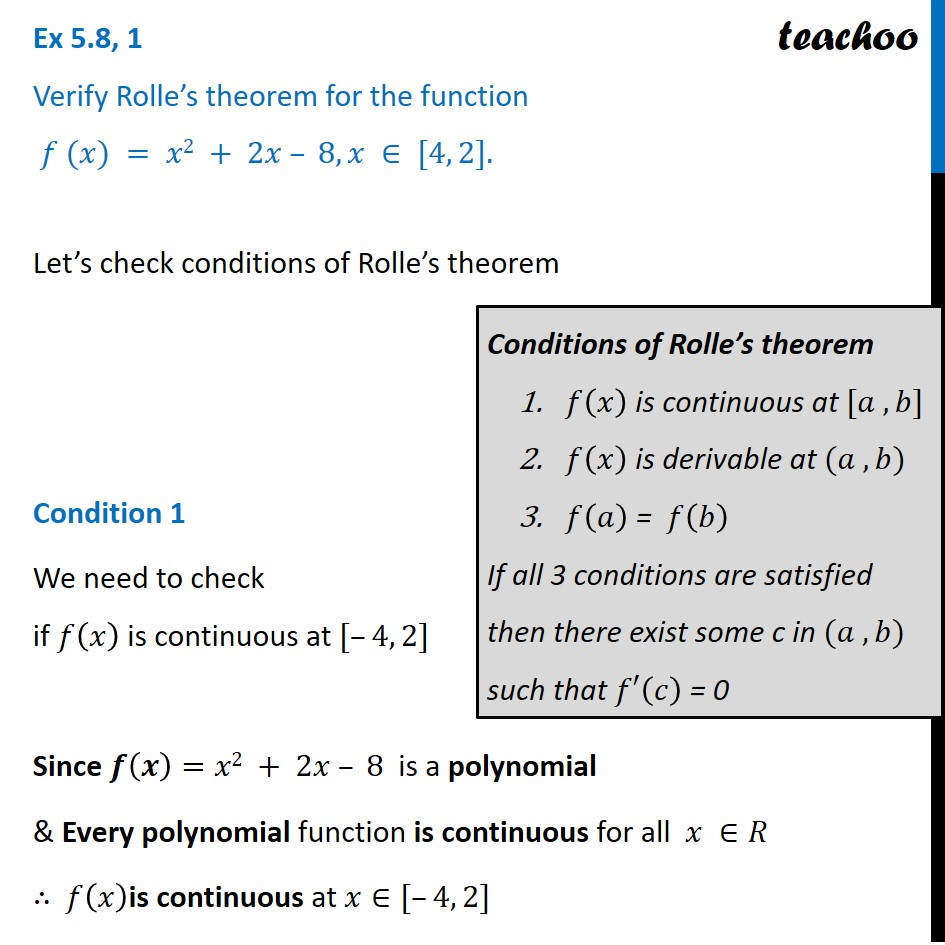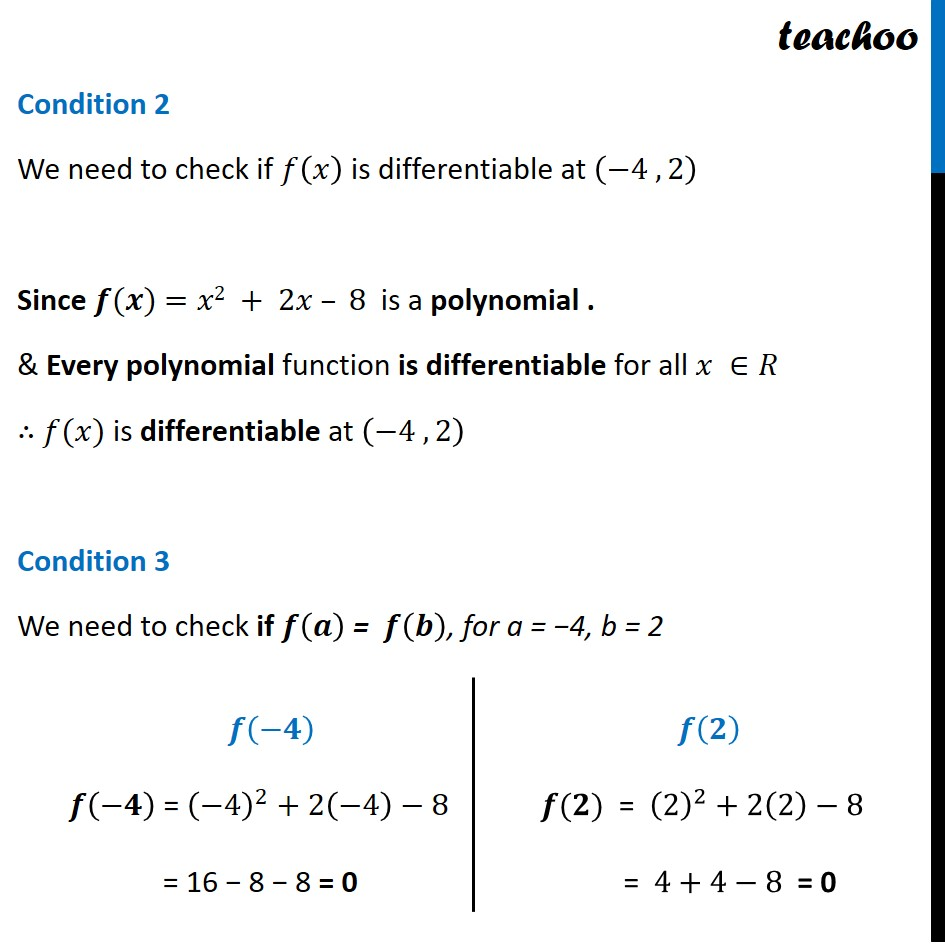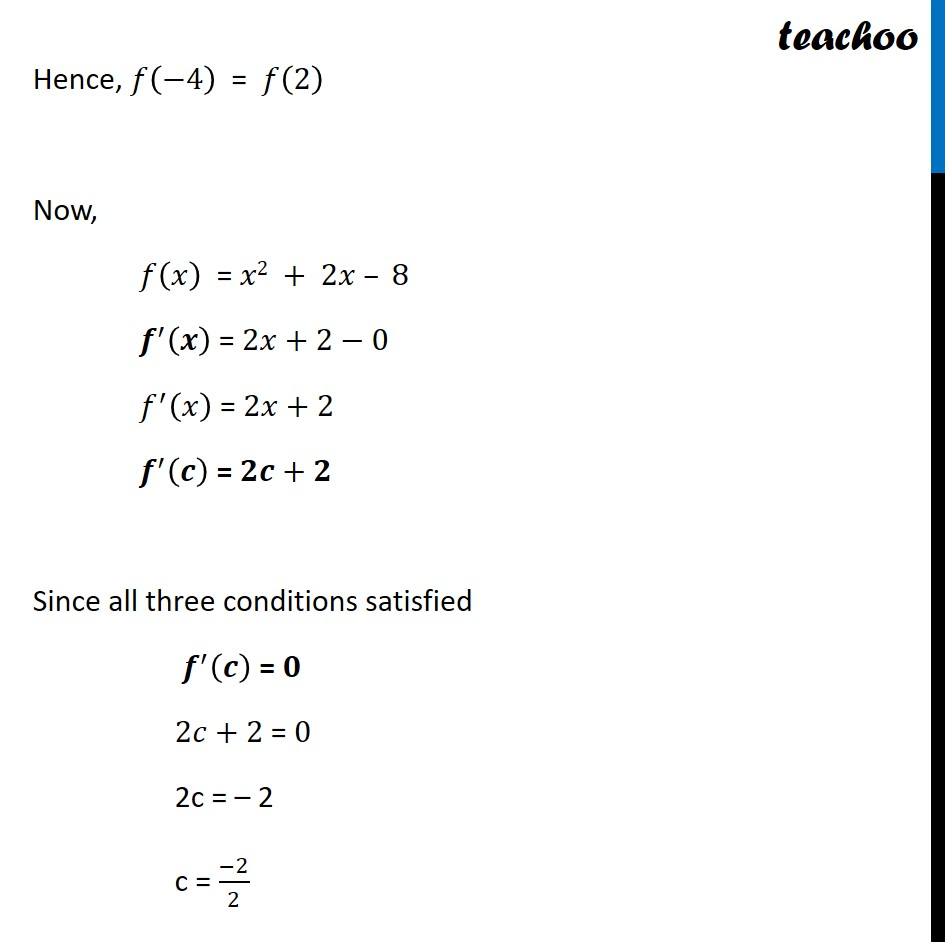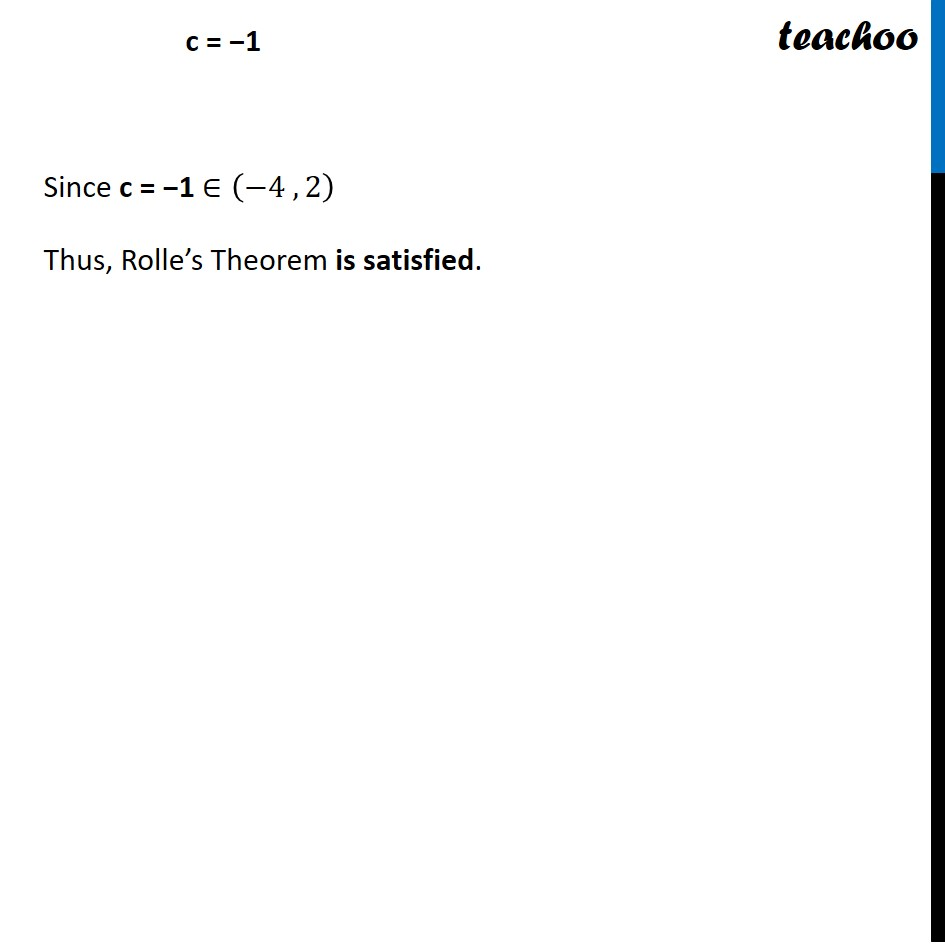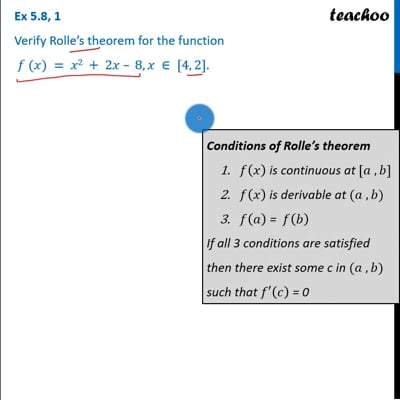This video is only available for Teachoo black users

Introducing your new favourite teacher - Teachoo Black, at only ₹83 per month

### Transcript

Ex 5.8, 1 Verify Rolle’s theorem for the function 𝑓 (𝑥) = 𝑥2 + 2𝑥 – 8, 𝑥 ∈ [4, 2].Let’s check conditions of Rolle’s theorem Condition 1 We need to check if 𝑓(𝑥) is continuous at [–4, 2] Since 𝒇(𝒙)=𝑥2 + 2𝑥 – 8 is a polynomial & Every polynomial function is continuous for all 𝑥 ∈𝑅 ∴ 𝑓(𝑥)is continuous at 𝑥∈[–4, 2] Conditions of Rolle’s theorem 𝑓(𝑥) is continuous at [𝑎 , 𝑏] 𝑓(𝑥) is derivable at (𝑎 , 𝑏) 𝑓(𝑎) = 𝑓(𝑏) If all 3 conditions are satisfied then there exist some c in (𝑎 , 𝑏) such that 𝑓′(𝑐) = 0 Condition 2 We need to check if 𝑓(𝑥) is differentiable at (−4 , 2) Since 𝒇(𝒙) =𝑥2 + 2𝑥 – 8 is a polynomial . & Every polynomial function is differentiable for all 𝑥 ∈𝑅 ∴ 𝑓(𝑥) is differentiable at (−4 , 2) Condition 3 We need to check if 𝒇(𝒂) = 𝒇(𝒃), for a = −4, b = 2 𝒇(−𝟒) 𝒇(−𝟒) = (−4)^2+2(−4)−8 = 16 − 8 − 8 = 0 𝒇(𝟐) 𝒇(𝟐)" = " (2)^2+2(2)−8" " "= " 4+4−8" = 0" Hence, 𝑓(−4) = 𝑓(2) Now, 𝑓(𝑥) = 𝑥2 + 2𝑥 – 8" " 𝒇^′ (𝒙) = 2𝑥+2−0 𝑓^′ (𝑥) = 2𝑥+2 𝒇^′ (𝒄) = 𝟐𝒄+𝟐 Since all three conditions satisfied 𝒇^′ (𝒄) = 𝟎 2𝑐+2 = 0 2c = – 2 c = (−2)/2 c = −1 Since c = −1 ∈(−4 , 2) Thus, Rolle’s Theorem is satisfied.